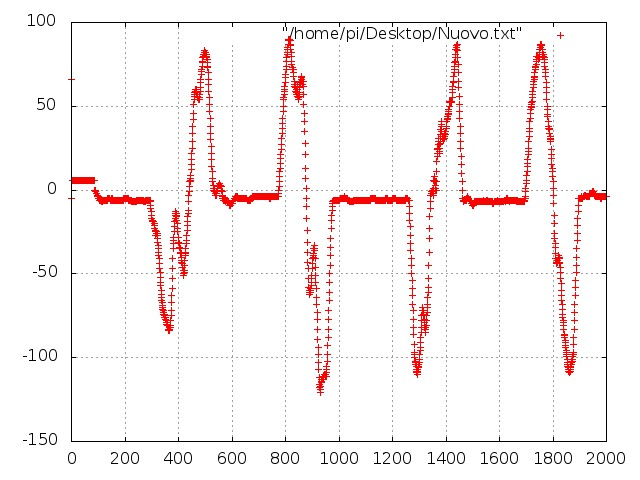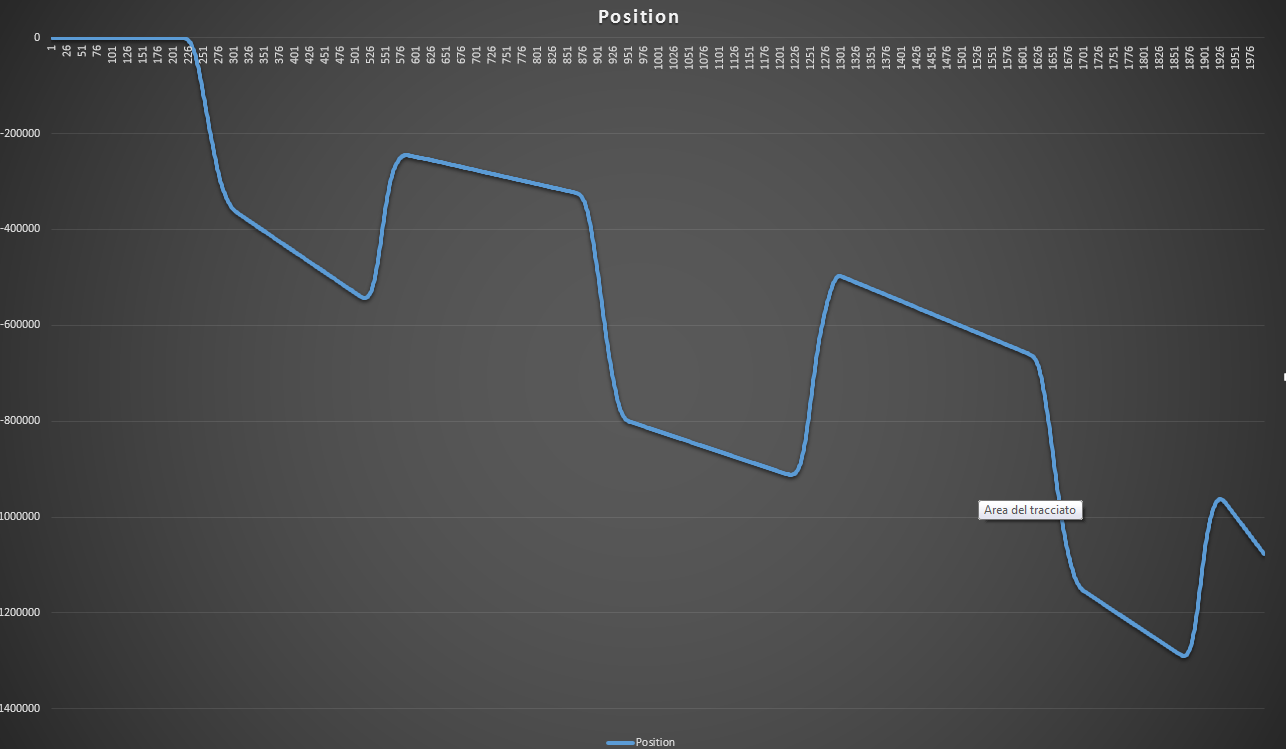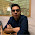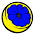## Thursday, July 25, 2013

### A simple dead reckoning algorithm on ArduinoAcceleration of an object moved from left to right, X4

I was trying to get the position of my accelerometer (relatively to its initial one) but googlein' around doesn't seem to be enough  this time. The concept is quite simple: since the acceleration is the first derivative of velocity, and position's second one, we could calculate the position of an object only by integrating (two times) the data from its acceleration. Ok, let's start from a good set of acceleration data. The graph above shows 2000 elements read out from the serial out of Arduino, with a rolling mean of 10 values. I'm trying to figure out why there are so many spikes to make it need 10 samples to get rid of them. Perhaps my "professional" solder process made some false-contact and the stirring of the circuits make them touch.

This is an example of this data loss (around the 925th sample) probably due to the phenomenon described above, or perhaps for the low quality of the contacts on the arduino side. Indeed I didn't use wires like these, but just a copper wire without its plastic cladding.
Anyway, let's do the dirty job. Since:

So, considering as a raw approximation a simple sum as the integrating function, we have that
1. velocity(i) = velocity(i-1) + acceleration (i)
2. position(i) = position (i-1) + velocity (i)
Whereas v(0)= p(0)= 0; For a first analysis I tried to implement the algorithm in Excel (offline, of course) and the partial results are show below. The blue line is the acceleration while the orange one is the velocity.Image 3

These are not from the same data as those in the first image, but the filtering is the same: a rolling mean of 10 values. The setup (Arduino + ADXL) is moved along the X-Axis for six times. Moreover, in this case I've already added a couple of further improvements. First, all the value below a threshold are thrown away to avoid the effect of the small vibrations. Second, since there could be an "offset" fixed value of static acceleration, this is calculated over a mean of 2048 samples and subtracted to all of the successive values.
Anyway we introduce an error at every step, which is integrated over time and so it keeps growing with the number of samples. If you click on the image 3 you can see how the velocity doesn't return to zero even between two movements!   The error in the estimation of the velocity brings to an even bigger error in the calculus of position:Image 4
Even if the object was moving alternatively between two points, it seems moving away from them (the system is not stable). Ok, so far so good. It could not be so simple!  :)
Let's try to reduce this error. If you have any advice, question, or if you just want to insult me, do it in the comments below. See you soon.

1.Excellent work there !
Would you mind sharing your code ?
I also need to calculate the number of steps,and by the way how did you decide the sensitivity to choose , I have an ADXL 335 offering +/- 3G , would that be enough for
getting coordinates and counting footsteps ?

2.Hi Jatin Batra! I'll add a link for the code as soon as I leave my office.
If you need to calculate the number of steps I think +/- 3G should be enough!
You could begin setting sensitivity to +/- 2G and try to jump, step, run, logging your data, and see if 2G are enough or you have to raise to 3G!
Keep me up date!

3.Thank you , can you please also expalin how this works

"considering as a raw approximation a simple sum as the integrating function, we have that
velocity(i) = velocity(i-1) + acceleration (i)
position(i) = position (i-1) + velocity (i) "

4.Do you mean "Why a simple sum can be considered as a integrating function?"
or "Why velocity(i) = velocity(i-1) + acceleration (i) and position(i) = position (i-1) + velocity (i) "

or both? :)

5.The later one , I do understand you are summing up discrete values from sensor (in this case accerelometer ) but how ?
I basically intend to find coordinates and steps of a user in a simple translatory motion (may be on a rough jerky arena) in uni-direction motion.
I see there is a time difference between mine and yours , where are you origrinally from ? :)

6.I'm from Italy!

Well... in my simple example I don't take in account the time between two values and I assume that

deltaT: (time(i) - time(i-1) ) = 1

So I simplify the equation

v(i) = v(i-1) + a(i) * deltaT

....writing...

v(i) = v(i-1) + a(i)

If you need to calculate more realistic values you have to multiplicate each value by your deltaT

7.8.